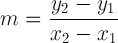×

Slope Intercept Form Calculator

Enter two points (x1, y1) and (x2, y2):
 x1: y1: x2: y2:

About Slope Intercept Form Calculator

The Slope Intercept Form Calculator is used to help you find the slope intercept form for the equation of the straight line that passes through two points. It also calculates the slope and the y-intercept of the line.

Slope Intercept Form

The slope intercept form for the equation of any straight line is given by:

y = mx + b

where:
m is the slope of the line
b is the y-intercept of the line

The slope m of the line through any two points (x1, y1) and (x2, y2) is given by:The y-intercept b of the line is the value of y at the point where the line crosses the y axis. Since for point (x1, y1) we have y1 = mx1 + b, the y-intercept b can be calculated by:

b = y1 - mx1

Watch Videos: